### Course description

This course covers eigenvalues and eigenvectors, first order ordinary differential equations,
ordinary linear differential equations of the second order, non-homogeneous equations with constant coefficients, Cauchy equation, power series solution of differential equations, Laplace transforms, line integral, complex analytic functions, series solution and systems of first order linear differential equations, basic concepts of Partial Differential Equations (PDE), techniques of solutions of first order PDE, Fourier series, second order PDE, vector and tensor analysis.

### Course outcomes

Upon the completion of this course, students will be able to:

• Find eigenvalues and eigenvectors of a given square matrix
• Distinguish various classes of differential equations
• State the underlying theory of linear ODEs
• Solve ODEs using various techniques
• Apply the theory of power series to solve certain classes of differential equations
• Apply Laplace transform to solve certain classes of ODE
• Solve system of differential equations
• Express physical problems in terms of differential equations
• Evaluate certain real integrals that are not accessible by real integral calculus
• State the fundamental theorem of line integrals
• Map regions conformally onto another
• Represent periodic functions by using Fourier series
• Solve PDE by using Fourier series
• Find covariant and contravariant components of a vector

### Course contents

Click the down arrow icon [ 🔽 ] to expand and collapse the course topics.

🔽 2 h 08 min | The Eigenvalue Problem
• Eigenvalues
• Eigenvectors
• Applications of eigenvalue problems
• Symmetric, skew-symmetric, and orthogonal matrices
• Diagonalization
🔽 1 h 15 min | Ordinary Differential Equations of First Order
• Basic concepts
• Geometric meaning of y’ = f(x, y)
• Direction fields
• Separable ODEs
• Exact ODEs
• Integrating factors
• Linear ODEs
• Bernoulli equation
🔽 1 h 02 min | Second Order Linear Ordinary Differential Equations
• Homogeneous linear ODEs with constant coefficients
• Euler-Cauchy equations
• Existence and uniqueness of solutions
• Wronskian
• Nonhomogeneous ODEs
🔽 1 h 40 min | Power Series Solution of Differential Equations
• Power series method
• Theory of the power series method
• Legendre’s equation
• Legendre polynomials
• Bessel’s equation
• Bessel functions
🔽 2 h 18 min | Laplace Transforms
• Laplace transform
• Inverse transform
• Linearity, s-Shifting
• Transforms of derivatives and integrals
• Unit step function, t-Shifting
• Convolution
• Integral equations
• Differentiation and integration of transforms
• Systems of ODEs
🔽 1 h 15 min | System of Ordinary Differential Equations
• Systems of ODEs
• Basic theory of systems of ODEs
• Constant-coefficient systems
• Phase plane method
• Criteria for critical points
• Stability
• Nonhomogeneous linear systems of ODEs
🔽 1 h 48 min | Complex Integration
• Line Integral in the complex plane
• Cauchy’s integral theorem
• Cauchy’s integral formula
🔽 1 h 04 min | Analytic Functions
• Derivatives of analytic functions
• Evaluation of line integrals
🔽 0 h 58 min | Conformal Mapping
• Conformal mapping
• Linear fractional transformations
• Special linear fractional transformations
🔽 0 h 48 min | Fourier Series
• Fourier series
• Functions of any period p = 2L
• Even and odd functions
• Half-range expansions
🔽 0 h 54 min | Vector and Tensor Analysis
• Introduction to tensor
• Symmetric and skew symmetric tensors
• Change of basis
• Reciprocal basis
• Covariant and contravariant components of a vector
🔽 1 h 10 min | Partial Differential Equations
• Basic concepts
• Modeling: vibrating string
• Wave equation
• Solution by separating variables
• Use of Fourier series
• D’Alembert’s solution of the wave equation and characteristics
• Heat equation

### This course includes: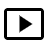14 h 30 min recorded video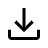Downloadable resources (books and articles)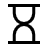One year access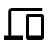Access on mobile and TV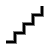Advanced Level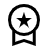Certificate of completion

#### Self-paced

8,900 Br
1 year of access
This course does not have any sections.